• PHP实现分页功能（一）上一页/下一页（含有分类功能的分页）
千次阅读
2020-09-17 16:03:50

以下方法是基于THINKPHP5框架开发，实现上一页/下一页按钮式的分页功能，如下图首先是简单的不涉及分类功能的分页

控制器代码

/*
*分页功能
* 参数：p 当前页码数
*      listpage 每页显示的数据条数
*      totalpage 总页数
* */
public function index(){
$p=input('p',1,'intval');//当前页码数，默认显示第一页$listpage=input('listpage',8,'intval');//每页显示的数据条数
$count=Db::name('shujubiao')->count();//数据总数$totalpage=ceil($count/$listpage);//总页数
$product=Db::name('shujubiao')->page($p,$listpage)->select();$this->assign('product',$product);$this->assign('p',$p);$this->assign('totalpage',$totalpage); return$this->view->fetch('页面');
}


前端代码，样式自行调试

<div class="page-box mt50 box flex_b">
<input type="hidden" id="page" value="{$p}"> <input type="hidden" id="totalpage" value="{$totalpage}">
<a href="javascript:;" class="page-item up">上一页</a>
<a href="javascript:;" class="page-item down">下一页</a>
</div>


js代码

//分页
//上一页
$(document).on('click', '.page-box .up', function() { var page =$("#page").val();
page--;
if (page < 1) {
page = 1;
return false;
}
location.href = "/模块名/控制器名/方法名/p/" + page + '.html';//跳转到自己的控制器方法
});
//下一页
$(document).on('click', '.page-box .down', function() { var page =$("#page").val();
var totalpage = $("#totalpage").val(); page++; if (page > totalpage) { page = totalpage; alert('已经是最后一页了'); return false; } location.href = "/模块名/控制器名/方法名/p/" + page + '.html';//跳转到自己的控制器方法 });  以上就可以实现简单的上一页/下一页功能； * * ## 分页功能通常会参杂分类功能，下面是此种样式下含有分类功能的分页代码。 控制器代码（和上面普通分页功能相比，只是添加了分类where条件） public function index(){ //参数 class_id 分类id$class_id=input('class_id','','intval');//参数@所属分类id
if(!$class_id){ //如果没有分类，显示全部商品 }else{$classidarr=[];
$classidarr=$class_id;
$classidarr=array_merge($classidarr,$this->loop($class_id));//所有下级分类id集合
$where['pclass_id']=array('in',$classidarr);//pclass_id为关联外键
}

//下面代码和普通分页代码基本一样，添加一个分类的where条件
$p=input('p',1,'intval');//当前页码数，默认显示第一页$listpage=input('listpage',8,'intval');//每页显示的数据条数
$count=Db::name('shujubiao')->where($where)->count();//对应分类下的数据总数
$totalpage=ceil($count/$listpage);//总页数$product=Db::name('shujubiao')->where($where)->page($p,$listpage)->select();$this->assign('product',$product);$this->assign('p',$p);$this->assign('totalpage',$totalpage);$this->assign('class_id',$class_id);//分类id return$this->view->fetch('页面');
}
/*
* 获得所有下级分类id
* 参数：$pid 上级分类id * */ private function loop($pid){
$idsarr=db('分类表')->where('pid',$pid)->column('id');
if($idsarr){ foreach($idsarr as $id){$idsarr=array_merge($idsarr,$this->loop($id)); } } return$idsarr;
}


前端代码，样式自行调试（和上面html代码相比，添加了分类参数的传递）

<div class="page-box mt50 box flex_b">
<input type="hidden" id="page" value="{$p}"> <input type="hidden" id="totalpage" value="{$totalpage}">
<input type="hidden" id="class_id" value="{$class_id}"> <a href="javascript:;" class="page-item up">上一页</a> <a href="javascript:;" class="page-item down">下一页</a> </div>  js代码（和上面js代码相比，添加了分类判断及参数传递） //分页 //上一页$(document).on('click', '.page-box .up', function() {
var page = $("#page").val(); var classid =$("#class_id").val();
if (!classid) {
classid = 0;
}
page--;
if (page < 1) {
page = 1;
return false;
}
location.href = "/模块名/控制器名/方法名/p/" + page+ "/class_id/" + classid+ '.html';//跳转到自己的控制器方法
});
//下一页
$(document).on('click', '.page-box .down', function() { var page =$("#page").val();
var totalpage = $("#totalpage").val(); var classid =$("#class_id").val();
if (!classid) {
classid = 0;
}
page++;
if (page > totalpage) {
page = totalpage;
return false;
}
location.href = "/模块名/控制器名/方法名/p/" + page+ "/class_id/" + classid + '.html';//跳转到自己的控制器方法
});


以上就可以实现与分类结合的上一页/下一页功能。
参数的传递方法不限，可根据自己要求进行修改调试。tp5分页
更多相关内容
• 用unity和php实现一个排行榜功能

注：此文章需配合以下文章一起使用
用unity和php实现一个排行榜功能（unity客户端篇）

## 开发环境安装

首先下载phpstudy，window64位版本
https://www.xp.cn/
安装，启动，打开localhost,看看是否正常

## 框架选用

选用thinkphp5这个框架，下载地址
https://www.thinkphp.cn/down/1278.html
解压，放到phpstudy的www目录下
打开localhost/public,即可看到thinkphp的欢迎页面

## 数据库准备

用navicat，新建数据数据库，我命名为untiy，unity库里新建数据表，命名自己定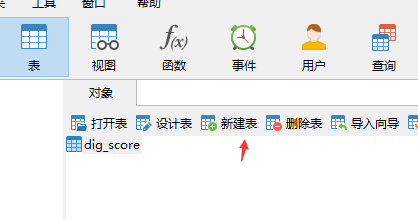表的字段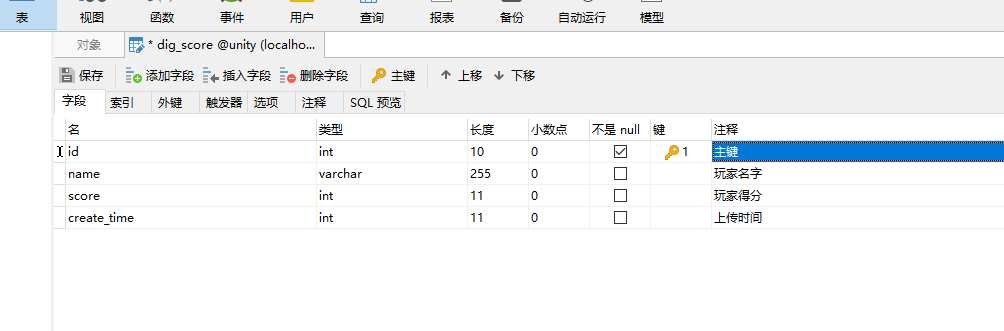thinkphp5配置mysql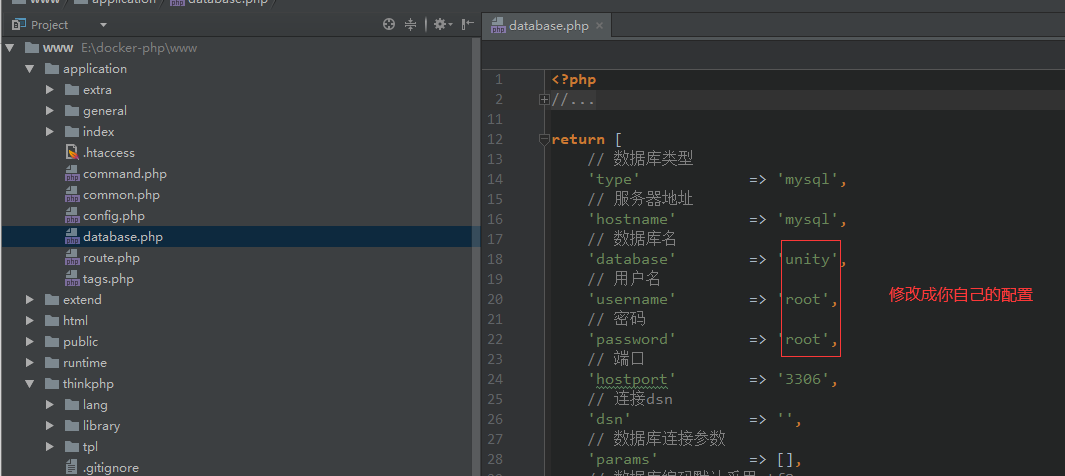根据这篇文章隐藏index.php,这样访问url的时候可以省略index.php
https://blog.csdn.net/nookl/article/details/80039996

## 生成模型

application目录下，右键打开命令行，键入以下命令，生成模型

php think make:model dig_score


## thinkphp5里排行榜数据读取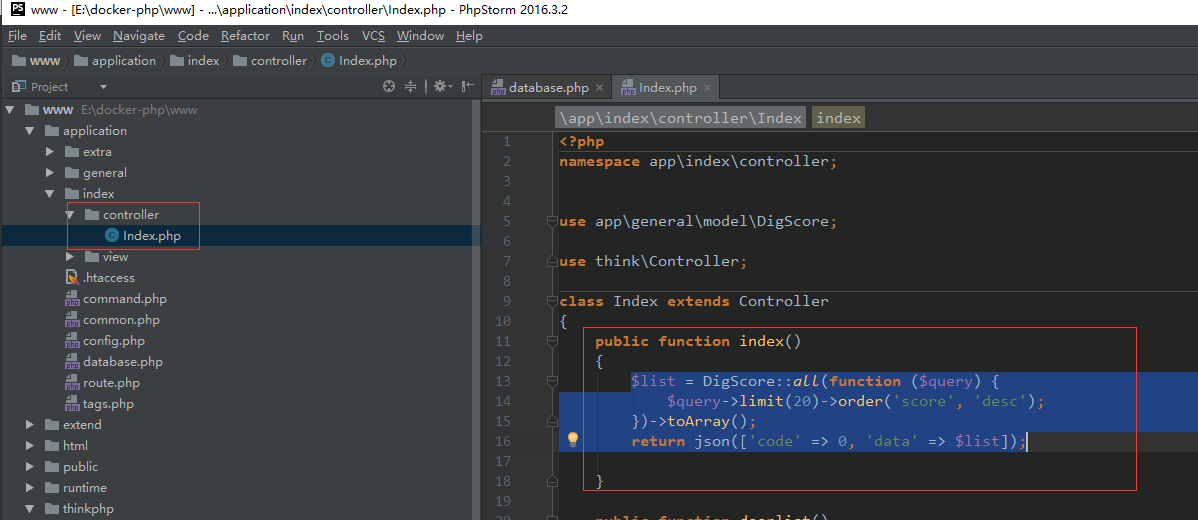$list = DigScore::all(function ($query) {
$query->limit(20)->order('score', 'desc'); })->toArray(); return json(['code' => 0, 'data' =>$list]);


这里取了分数最高的前20人，其他数字请自行修改limit

navicat里双击数据表，手动写入几条数据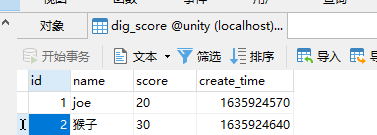此时访问localhost/public,就能看到json格式的排行数据了，这个json数据就是unity那边的排行榜展示来源了

在index方法下面，准备以下提交排行分数的代码

 public  function addscore(){
if(empty($_POST)){ return json(['code'=>1,'data'=>'缺少参数']); }$score = new DigScore();
$score->score =input('?post.score');$score->name = input('?post.name');
$score->create_time = time();$score->save();
return json(['code'=>0]);
}


打开tp5，application下的route.php文件
粘贴以下代码(访问url时更简短)

Route::post('addscore','index/index/addscore');


至此，服务端已经准备完毕，可以开始unity客服端代码编写

展开全文unity c# 游戏开发
• ## PHP实现留言板功能

万次阅读 多人点赞 2019-04-17 21:52:49
作为一个PHP的初学者，我试着写了一个留言板，页面有点丑，多多见谅，嘻嘻嘻 #我们写留言板需要用到数据库，所以我们先要建立三个表 user表 #首先需要写一个注册与登录 ##注册 zhuce.html <meta charset=...

作为一个PHP的初学者，我试着写了一个留言板，页面有点丑，多多见谅，嘻嘻嘻
#我们写留言板需要用到数据库，所以我们先要建立三个表
user表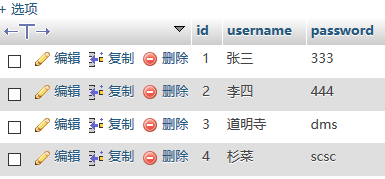friend表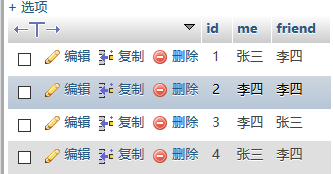text表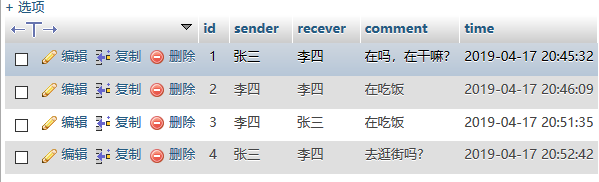#首先需要写一个注册与登录

##注册
zhuce.html

<meta charset="utf-8">
<title>zhuce</title>
<body>
<form method="POST" action="zhuce.php">
<div style="margin-left: 500px;margin-top:200px;height: 250px;width: 250px">
<h1>用户注册页面</h1>
<div><input type="submit"  name="submit" value="注册"></div>
</div>
</form>
</body>



zhuce.php

<?php
session_start();
$user=$_POST["username"];
$pwd=$_POST["password"];
if($user==""||$pwd=="")
{
}
else
{

$link=mysqli_connect("localhost","root","","liuyan");//连接数据库 mysqli_query($link,"set names utf8");
$sql="select username from user where username='$_POST[username]'";
$result=mysqli_query($link,$sql);//执行sql语句$num=mysqli_num_rows($result);//统计执行结果影响的行数 if($num)//如果存在该用户
{
}
else//注册新用户
{
$sql_insert="insert into user (username,password)values('$_POST[username]','$_POST[password]')";$res_insert=mysqli_query($link,$sql_insert);
if($res_insert) { echo "<script>alert('注册成功！');window.location='denglu.html';</script>"; } else { echo "<script>alert('系统繁忙请重试！'); history.go(-1);</script>"; } } } ?>  #效果如下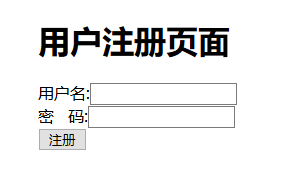##登录 denglu.html <head> <meta charset="utf-8"> <title>denglu</title> </head> <body> <form method="POST" action="denglu.php"> <div style="margin-left: 500px;margin-top:200px;height: 250px;width: 250px"> <h1>用户登录页面</h1> 用户名:<input type="text" name="username"> <div>密&nbsp;&nbsp;&nbsp;码:<input type="password" name="password"></div><br/> <input type="submit" name="submit" value="登录"> <a href="zhuce.html">注册</a> </div> </form> </body>  denglu.php <?php session_start();$user=$_POST["username"];$_SESSION["uesrname"]=$user;//session超全局变量$pwd=$_POST["password"];//获取密码 if($user=""||$pwd="") { echo "<script>alert('请输入用户名或密码！'); history.go(-1);</script>"; } else {$link=mysqli_connect("localhost","root","","liuyan");//连接数据库
mysqli_query($link,"set names utf8");$sql = "select username,password from user where username = '$_POST[username]' and password = '$_POST[password]'";
$result=mysqli_query($link,$sql);//执行sql语句$num=mysqli_num_rows($result);//统计影响结果行数，作为判断条件 if($num)
{
}
else
{
}
}
?>


#效果如下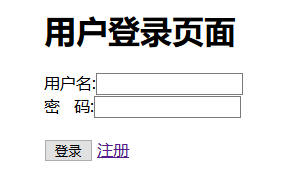#下面需要我们写一个登录进去的页面，它显示别人给你发来的留言以及进入你要给别人留言的页面或者退出系统，在这里我将html代码嵌入php
php代码如下
003.php

<?php
session_start();
global  $user;//定义$user
global $user1;$_SESSION["username"]=$user;$username=$_SESSION["uesrname"];$user1=implode("",$_SESSION);//将session中的数组变为字符串元素$link=mysqli_connect("localhost","root","","liuyan");//连接数据库
mysqli_query($link,"set names utf8");$sql="select * from text where recever='{$username}'";$result=mysqli_query($link,$sql);//执行语句
if($num=mysqli_num_rows($result))//将HTML嵌入PHP中，实现从数据库中获得留言信息
{?>
<!DOCTYPE html>
<html>
<meta charset="utf-8">
<body>
<div>
<a href="fabu.html">发布信息</a>&nbsp;&nbsp;&nbsp;&nbsp;&nbsp;&nbsp;
<a href="tuichu.php">退出系统</a></h3></div>
<br/><br/>

<h2>留言信息:</h2>
<tr bgcolor="#8BBCC7">

<td>发送人</td>
<td>接收人</td>
<td>发送时间</td>
<td>信息内容</td>
<?php echo '<pre>';
while($row=mysqli_fetch_array($result,MYSQLI_ASSOC))
{?>
<tr bgcolor="#FFFFFF">
<td ><?php echo $row['sender'];?>&nbsp;</td> <td >&nbsp;<?php echo$row['recever'];?>&nbsp;</td>
<td >&nbsp;<?php echo $row['comment'];?>&nbsp;</td> <td >&nbsp;<?php echo$row['time'];?>&nbsp;</td>
<?php
}
?>
</tr>
</table>
</body>
</html>
<?php

}?>


#效果如下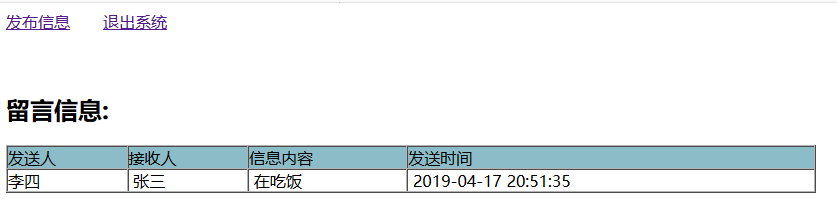#接下来我们就该写发布的页面以及PHP代码了
fabu.html

<head>
<meta charset="utf-8">
<title>fabu</title>
<body>
<form  method="POST" action="fabu.php">
<h1>发布信息<h1>
<a href="003.php">主页面</a>
<div>接收人:<input type="text" name="recever">
</select>
</div>
</select>
</div>
<br/>
<div>
信息内容:<textarea input type="text" name="neirong"></textarea>
</div><br/>
<input type="submit" value="发送">
</form>


fabu.php

<?php
session_start();
global $user;$re=$_POST["recever"];//获取recever$comment=$_POST["neirong"];//获取留言 @date_default_timezone_set(PRC);//将数组变为字符串函数$time=date("Y-m-d G:i:s");//获取时间，G为24小时制
$_SESSION["username"]=$user;//开启session
$user1=implode("",$_SESSION);//将数组转为字符串
$link=mysqli_connect("localhost","root","","liuyan");//连接数据库 mysqli_query($link,"set names utf8");
$sql="insert into text(sender,recever,comment,time) values('$user1','$re','$comment','$time')";$result=mysqli_query($link,$sql);//执行语句
$sql1="insert into friend(me,friend) values('$user1','$re')";//将me，friend存入数据库$result=mysqli_query($link,$sql1);//执行语句
if($recever=""||$comment="")
{
}
else
{
}
?>


#效果如下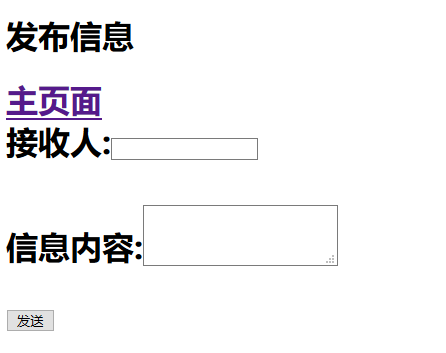#最后是退出系统

<?php
session_start();
unset($_SESSION["uesrname"]); echo "<script>alert('退出成功！');window.location='denglu.html';</script>"; ?>  #效果如下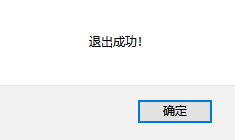##第一次写博客，写得不好多有见谅，希望对大家学习PHP有所帮助，如有疑问或者改进方法请留言。 展开全文MYSQL数据库 • 上一页和下一页的代码实现：$prev = $curPage - 1 1 ? 1 :$curPage - 1 ; $pageNumString .= "<li><a href='listNews.php?curPage=$prev '>«</a></li>" ; $pageNumString... 我们经常在网上看到分页的出现，最近没事。看看公司的后台代码，对这个小模块进行封装。制作成一个函数。现在将自己的这个思考过程，写下来。 首先我们来看看分页的逻辑：首先我们要确定首页和尾页的限制，首页必须限定在1，尾页限制的数字，需要结合我们的数据库的数据条数，和每页的显示数量。尾页=ceil（数据库总条数/每页显示的数量），向上取整。这就是我们尾页的限制数字。接下来我们就要控制数字出现的规律了。如图所示：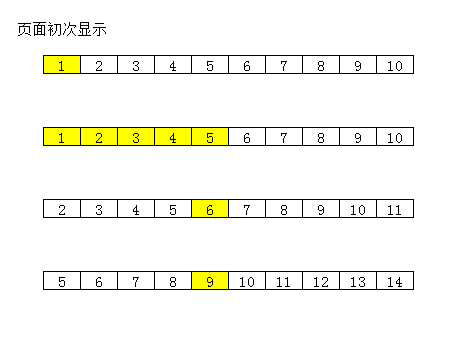如果我们设定显示10页，那么当我们点击页码，当前页小于或者等于5页的时候，是一直停留在10页的位置（假设尾页没有限制的情况下），当点击的页数大于5时，随着我们的点击，页码也在往后推移，于是就出现了下面的规律，尾页=当前点击也+5，首页为尾页-9.通过这个规则我们可以书写出尾页和首页的范围。$totalpage为我们总页数的显示。也就是尾页的数量。

    if($curPage <=5){$begin =1;
$end =$totalpage>=10?10:$totalpage; }else{$end = $curPage +5>$totalpage?$totalpage:$curPage +5;
$begin =$end -9<=1?1:$end -9; } 我们获得上面的规则就是分页的核心。然后当前点击的页码，需要特别标注一下，也就是当，当前页==我们的循环页码一项时，出现特殊标注。其他正常显示： for($i =$begin;$i <=$end;$i ++){
//使用if实现高亮显示当前点击的页码
if($curPage ==$i){
$pageNumString .= "<li class='active'><a href='listNews.php?curPage=$i '>$i </a></li>"; } else{$pageNumString .= "<li><a href='listNews.php?curPage=$i '>$i </a></li>";
}
}
如上面的所示，这样我们就做到了点击当前页，当前页码特殊标注。剩下的事情就是我们完善一下。上一页和下一页的代码实现：

$prev =$curPage - 1 <= 1? 1:$curPage - 1;$pageNumString .= "<li><a href='listNews.php?curPage=$prev '>&laquo;</a></li>";$pageNumString .= "<li><a href='listNews.php?curPage=$next '>&raquo;</a></li>";$next = $curPage + 1 >=$totalpage?$totalpage:$curPage + 1;
点击的时候自动加一和减一。同时对页码进行限制，防止超出我们规定的范围。

$pageNumString .= "<li><a href='listNews.php?curPage=1'>首页</a></li>";$pageNumString .= "<li><a href='listNews.php?curPage=$totalpage '>尾页</a></li>"; 上面我们把首页和尾页对应的两个极限值写入代码中。对于代码每页显示的数量，我们需要使用SQL语句的查询的时候进行限制： //人为的定义一个最大的页码数$rowsPerPage = 10;
//获得数据
$offset = ($curPage - 1) *$rowsPerPage;$sql = "select * from news order by n_sort desc limit $offset ,$rowsPerPage ";
$return = mysqli_query($obj,$sql); //获取所有的数据 主要是limit语句的使用。对于每页显示的数量，进行筛选。再次通过SQL语句查出所有的数据总数： //获得数据总条数$sql = "select count(*) as total from news";
$resq = mysqli_query($obj,$sql);$arr = mysqli_fetch_assoc($resq);$totalRows =$arr[ 'total']; 然后整个的逻辑我们走了下来，剩余的就是封装函数了，对于其中我们可以确定的是那些变量是参数：比如总页数，每页显示的数量，当前页码。三个变量作为函数的形参。接下来我们看看效果图：我们布局使用了 Bootstrap的布局样式：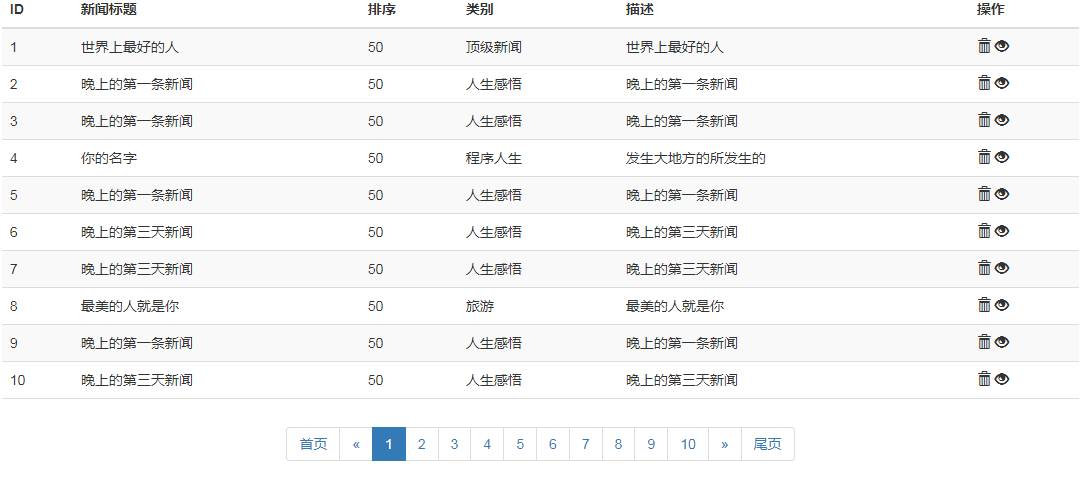上述就是我们的思考过程，附上所有的代码： //当前页码数，默认当前页码为1$curPage = isset($_GET[ 'curPage'])?$_GET[ 'curPage']: 1;

//获取所有的新闻数据

$obj = mysqli_connect( '127.0.0.1', 'root', '151766'); //设置字符集$sql = "set names utf8";
$return = mysqli_query($obj,$sql); //选择数据库$sql = "use php1210";
$return = mysqli_query($obj,$sql); //人为的定义一个最大的页码数$rowsPerPage = 10;
//获得数据
$offset = ($curPage - 1) *$rowsPerPage;$sql = "select * from news order by n_sort desc limit $offset ,$rowsPerPage ";
$return = mysqli_query($obj,$sql); //获取所有的数据 //获得数据总条数$sql = "select count(*) as total from news";
$resq = mysqli_query($obj,$sql);$arr = mysqli_fetch_assoc($resq);$totalRows =$arr[ 'total'];$totalpage = ceil($totalRows /$rowsPerPage);
//存储页码字符串
$pageNumString = ""; if($curPage <= 5){
$begin = 1;$end = $totalpage >= 10? 10:$totalpage;
} else{
$end =$curPage + 5 >$totalpage?$totalpage:$curPage + 5;$begin =$end - 9 <= 1? 1:$end - 9;
}
//实现上一页
$prev =$curPage - 1 <= 1? 1:$curPage - 1;$pageNumString .= "<li><a href='listNews.php?curPage=1'>首页</a></li>";
$pageNumString .= "<li><a href='listNews.php?curPage=$prev '>&laquo;</a></li>";

//根据起始页与终止页将当前页面的页码显示出来
for($i =$begin;$i <=$end;$i ++){ //使用if实现高亮显示当前点击的页码 if($curPage == $i){$pageNumString .= "<li class='active'><a href='listNews.php?curPage=$i '>$i </a></li>";
} else{
$pageNumString .= "<li><a href='listNews.php?curPage=$i '>$i </a></li>"; } } //实现下一页$next = $curPage + 1 >=$totalpage?$totalpage:$curPage + 1;
$pageNumString .= "<li><a href='listNews.php?curPage=$next '>&raquo;</a></li>";
$pageNumString .= "<li><a href='listNews.php?curPage=$totalpage '>尾页</a></li>";

与所有的前端和后端爱好者共勉.....

展开全文分页
• （1）前端html+css页面(2)php连接数据库（3）数据库增删改查数据库 mysql
• 通过form标签实现表单功能 实现php与mysql数据库交互 使用数据库语句对记录进行操作 任务方法 form标签构造表单 mysqli_connect() 函数连接数据库 mysqli_query() 函数执行数据库语句 注： 在实现注册功能时:...mysql
• 今天对之前的用户管理模块实现了一个简单的分页功能，大致样式如下： 接下来谈谈具体实现的步骤： 1、既然涉及到分页，首先肯定是从数据库获取的数据就有限制，这个限制就是用limit，比如下面这个sql语句： \$...mysql
• 说到使用PHP进行网站开发，自然离不开各种PHP的开发框架，开发框架为我们提供了...那么这一篇博文，我们就先来一个简单点的功能，也就是说说MVC框架的路由功能实现方法。  一般的单入口框架路由都是这样的结构：lomvc
• 今天域名备案终于好了，开始搞第二步，实现直播功能，移动端可以参考七牛云SDK，下面是服务端推流案例，本次使用的是rtmp流实现直播，在控制台找到直播云服务，创建直播云空间 创建好直播空间后会生成几个二级域名...
• ## PHP实现翻译功能

万次阅读 2015-12-28 21:16:12
今天我们聊聊用PHP实现翻译功能，当然PHP这门语言它肯定无法成为一个翻译家，我们需要借助专门做翻译这 件事情的程序给我们开放的API接口来获取数据，最终交由PHP来解析数据，展示到我们需要的平台上。  大家应该都...开发API HTTP 数组 对象
• PHP实现创建以太坊钱包、转账等功能 解决：php如何调用以太坊JSON-RPC接口创建钱包 准备：（一样都不能少） 1.Geth下载（对以太坊了解不深的可以后面自己查找有关geth的资料，与此类似的客户端还有Ethereum、Parity ...geth json-rpc
• 技术流人员都开始研究各种红包算法，别看一个简单的红包功能看起来(说起来)简单，但是实现起来真不简单。首先我们不光要考虑到生成各个红包金额值的合理性(所有红包值不能大于总数)，还要考虑各个类型的抢红包模式...
• Vim配置#Vim中实现php代码自动完成，提示功能Vim页面静态化
• 是服务器后端接口，用于接收小程序传过去的关键词的，下面会有这个后端PHP文件。 const app = getApp() Page({ data: {}, //执行点击事件 formSubmit: function (e) { //声明当天执行的 var that = this; //获取表单...搜索 小程序搜索
• ## PHP实现购物车功能

千次阅读 2012-07-13 00:32:43
网上购物现已成为时尚，客户选择一个商品将其放入到购物车，然后返回继续购物或者去收银台，这个功能如何实现呢？今天capucivar就将使用PHP实现这个购物车的功能。 首先，做一个简单的首页，从数据库中查询出来几...session mysql border
• 微信小程序有一个非常强大的分享转发功能，能够让优秀的小程序在朋友圈里迅速传播，并通过用户之间的互动实现砍价等功能。之前做了一个名为臻美酒行的小程序，做了普通购物、团购、砍价功能。其中，团购和砍价都用到...
• 网站的安全性是开发者不可忽视的一个问题，目前使用最多的一种可以提高网站安全性的...下面就为大家详细解释验证码功能机制的实现思路以及简单的实现方法。 1、验证码功能机制实现思路 ① 常规的验证码实现验证码
• PHP 　PHP（Hypertext Preprocessor）是一种嵌入HTML页面中的脚本语言。它大量地借用C和Perl语言的语法， 并结合PHP自己的特性，使Web开发者能够快速地写出动态产生页面。 　PHP是完全免费的开源产品，不用花钱，...
• ## Php实现验证码功能

千次阅读 2014-09-23 16:23:03
验证码这样的功能可以说是无处不在了，接下来使用php实现验证码这样的功能，这里我是将验证码实现抽取到一个类中独立开来，那么后面如果再使用到验证码功能，直接引入该类文件并创建该类的实例，就可以使用验证码...验证码
• 因此，现在该让我们深入研究一些最令人兴奋的新增功能和新功能，这些功能将使PHP更快，更可靠。 。 实际上，即使PHP 7.4显着提高了性能并提高了代码的可读性，PHP 8仍将是PHP性能的真正里程碑，因为JIT包含的建议已...
• ## php调取摄像头实现拍照功能

千次阅读 热门讨论 2018-11-09 18:40:22
最近做的商户后台要实现调取摄像头拍摄用户打卡照片的功能，找资料研究了下，终于黄天不负有心人，成功了，下面我分步骤将代码贴出来，希望能有帮助 代码有点多，但是每一步都很好理解，首先是HTML代码，写一个form...
• PHP实现word（office文件）转PDF的功能并添加水印 开发目的：用PHP实现在linux服务器上在线批量转换office文件成PDF文件，常应用于文件管理较多的后台管理系统。 技术选择： 【PHP+OpenOffice+FPDI+FPDF】 首先要...
• 关于PHP发送邮箱验证码功能介绍   PHP语言发送邮箱验证码，可以使用PHPMailer这个现成的类文件，完美集成实现邮箱发送验证码   前期准备:a).PHPMailer下载地址：在git上获取最新版即可：...
• 一般类似oa或者crm等管理系统可能都会遇到需要再线查看word文档的功能，...今天看到网上一遍文章《LINUX下面PHP文件转换》，介绍怎么样在linux下使用Openoffice 3 , Pdf2Swf tool , Jodconverter , FlexPaper，实现文档openoffice
• ## Redis实现秒杀功能

万次阅读 2019-04-17 18:44:21
Redis使用watch完成秒杀抢购功能： redis使用watch完成秒杀抢购功能： 使用redis中两个key完成秒杀抢购功能，mywatchkey用于存储抢购数量和mywatchlist用户存储抢购列表。它的优点如下： 1.首先选用内存数据库来抢购...Redis
• 目标：我希望通过套接字的TCP传输来搭建一个服务器，这个服务器的作用是：接受多个客户端的连接并完成他们的相互通信。比如客户端A，客户端B同时连接到服务器S，客户端A向服务器S发送消息，服务器S会将A的消息转发给...socket windows
• PHP个人相册管理系统，实现了相册分类和相册分组，还有相册图片管理，数据库采用mysql，实现了登录注册功能PHP个人相册源码，相册管理系统，PHP相册管理系统，界面美观简单，适合用来当做课程设计或者简单毕业设计...数据库 mysql...